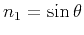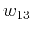On anelliptic approximations for qP velocities in TI and orthorhombic mediaNext: Muir and Dellinger Approximations Up: Transversely isotropic media Previous: Transversely isotropic media

## Exact Expression

The phase velocity of qP waves in TI media has the following well-known explicit expression (Gassmann, 1964; Berryman, 1979):(9)

whereare density-normalized stiffness tensor coefficients in Voigt notation,,, andis the phase angle (measured from the vertical axis). Group velocity can be determined from phase velocity using the general expression (Cervený, 2001)(10)

wheredenotes the identity matrix,is the phase direction vector, andis the gradient ofwith respect to. Using Muir-Dellinger parameters, the exact phase velocity for qP waves (equation 9) can be expressed as(11)

whereOn anelliptic approximations for qP velocities in TI and orthorhombic mediaNext: Muir and Dellinger Approximations Up: Transversely isotropic media Previous: Transversely isotropic media

2017-04-14